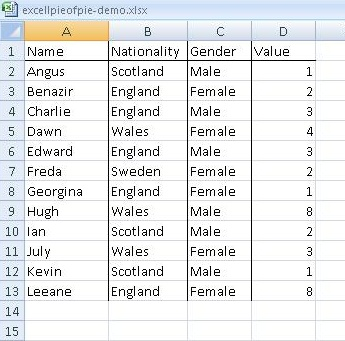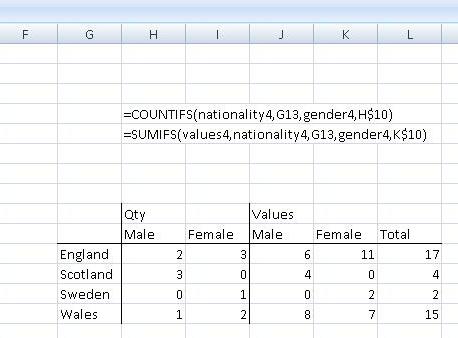In some of the more complex spreadsheets I have built recently I have wanted to select data from a table based on two conditions. This can be done using the functions COUNTIFS() and SUMIFS(). Once again using range names helps. These functions offer one the the equivilent of boolean AND, and they can be made to implement OR as well.

I have built an example which is in the tab named IFS in my example spreadsheet, which also contains the examples from my previous articles about excel.

My sample data set consists of a table, with our friends who have a key of their name. Each person has a nationality, a gender and a value column.I have then built a summary table using the two functions. For COUNTIFS(), the syntax consists of pairs of a ranges and a value. The ranges can be names of ranges or explicit rnage declarations and the value can be a cell or a value. (I have used the UNIX shell || as an “or” in the example below.

=COUNTIFS(nationality4, \${cellcontaining a value}||Value)

the range, value pairs can be repeated as they are in the example file.

Going back to our example data table, see above, in order to add the value column according to two criteria, for example in order to know the (nationality,  gender, sum(value)) matrix, you need SUMIFS(). This is similar to COUNTIFS() but preprends the summed column to the range value pairs, so

=SUMIFS(values4,  nationality4, \${cellcontaining a value}, gender4, \${cellcontaining a value})

The data results and example syntax are shown below.To explain the syntax strings, the values in the range/value pairs are explict cell references. Column G is the list of Countries on the left of the table and row 10 contains the Male/Female values.

These functions implement logical AND, if you want OR functions you’ll need to add two expressions together, but see below and if you want to implement NOT, then you’ll need to subtract. If you want an exclusive OR, i.e the sum of those cases which meet condition 1 or condition2 then you will need to add the two sums together and subtract those cases which meet both criteria.

Sum(Value, OR) = sumifs(V, R1,C1) + sumifs(V,R2,C2) – sumifs(V, R1,C1,R2,C2)

This is because those cases which meet both criteria will be in both the first two sums. If you wanted a true exclusive OR, then you should subtract two times the value of the final function so as to subtract the cases where both C1 & C2 are true from both the first and second function. Some with more recent mathematics training could probably make something of that.

These functions are not available in my version of Open Office V3.2.1 I obviously need to move forward to Libre Office, the check for updates on my OO install failed. Sigh! The Open Office file has been updated and is available here….

There is a Microsoft Help page on SUMIFS.

Selecting from an excel array using two or more criteria

This site uses Akismet to reduce spam. Learn how your comment data is processed.